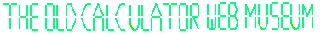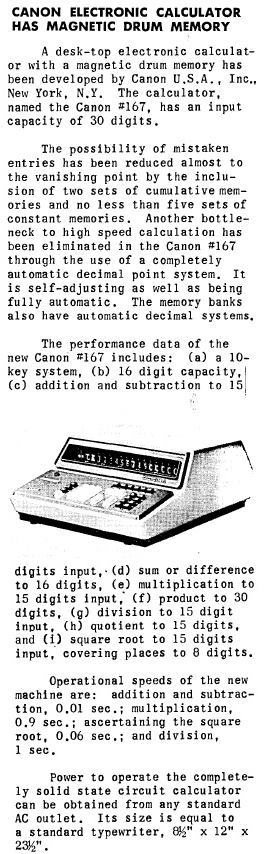+Home Museum Advertising News Archive Articles EMail

News Archive - Canon 167 IntroductionCanon 167 Announcement
Computers and Automation, September, 1967

Note that the article very likely contains a typographical error in the operational speed quoted for the square root operation. The article states a time of 0.06 second which is extraordinarily fast for a more complex operation like square root. The correct timing is likely to be 0.6 second, or 600 milliseconds. The square root is calculated to only 8 digits behind the decimal point. Even with the limited precision and assuming a very efficient square root algorithm, there is no way a machine that adds and subtracts in 10 milliseconds can possibly complete a square root calculation in only 60 milliseconds. Digit-by-digit calculation of square roots using the efficient Sum of Odd Integers method is very similar to division, performing numerous subtraction operations to arrive at a result. Division is quoted as taking up to 1 second with a full 15 digits of quotient, so a time of 600 milliseconds makes much more sense to perform a square root operation with limited precision as compared to division. It's likely that the extra zero behind the decimal point in the quoted time of 0.06 seconds was simply a typo when the article was prepared, and not caught in editing. The "Sum of Odd Integers" algorithm processes the radicand two digits at a time, thus, with a radicand of up to 15 digits, only a maximum of eight cycles through the algorithm would be required, which is why the square root operation would take less time than a division, which must process each digit of the divisor one at a time.